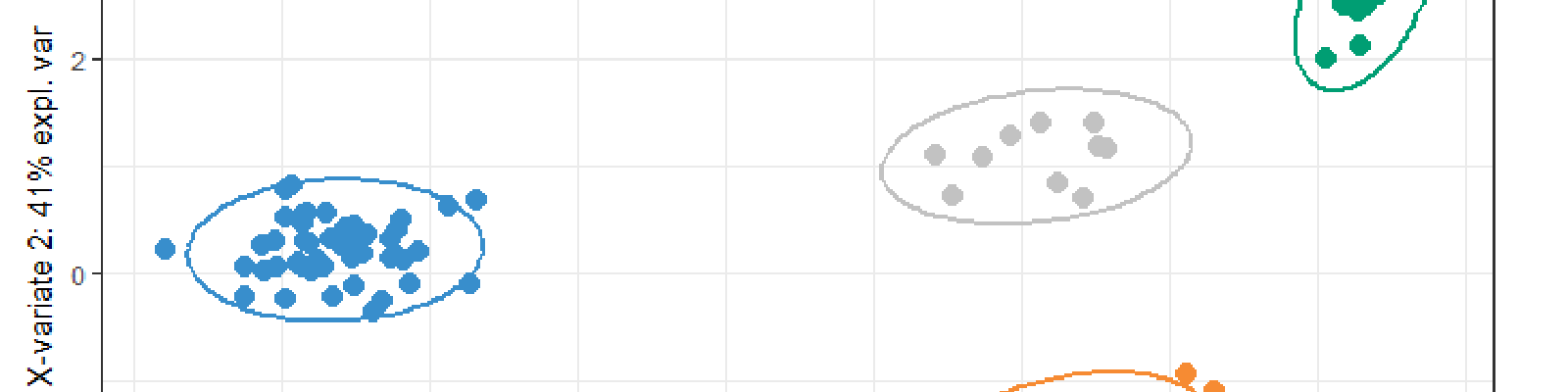Simulated datasets analysed with PLS under discriminant analysis

# Overview

The PLS tool is powerfull to tackle the curse of dimensionality, especially if the dataset suffers form high colinearities or if $n<p$. It even works when the response matrix is multivariate!

That course, given to M2-biostatistics students of ISPED, website introduces that tool and its sparse version thanks to which this is easily possible to select variables.

# Magistral course

• Recalls over linear regression and non-invertible cases,

• PLS1 and PLS2 algorithms.

• sPLS from the Lasso point of view.

• A case study

# Practical work on R

• sPLS-DA on simulated datasets: A 4-classes discrimination simulated problem to show the importance of the cross-validation process for supervized problems rather than explained variance

• sPLS-DA for single-cell 10X dataset: The application of the PLS method to a Discriminant Analysis case for Single-cell 4 classes discrimination:

• Statement
• Correction
• sPLS regression: The classical application to the sPLS regression model to the liver.toxicity dataset:

• Statement
• Correction